EE - 451 / E01 spring 2003

Professor: Conner

Submitted By:

Andrew Buettner

Lab #3: Digital Modulation and Demodulation

Sunday, May 04, 2003

1) Cover Page 1

3) Objective 5

4) Components Used 5

5) Procedures 5

6) Lab Data / Results 5

1) Table 1 5

2) Diagram 1 6

3) Diagram 2 7

4) Diagram 3 7

5) Diagram 4 8

6) Diagram 5 8

7) Diagram 6 9

8) Diagram 7 9

9) Diagram 8 10

10) Diagram 9 10

11) Diagram 10 11

12) Diagram 11 11

13) Diagram 12 12

14) Diagram 13 12

15) Diagram 14 13

16) Diagram 15 13

17) Diagram 16 14

18) Diagram 17 14

19) Diagram 18 15

20) Diagram 19 15

21) Diagram 20 16

22) Diagram 21 16

23) Diagram 22 17

24) Diagram 23 17

25) Diagram 24 18

26) Diagram 25 18

27) Diagram 26 19

28) Diagram 27 19

29) Diagram 28 20

30) Diagram 29 20

31) Diagram 30 21

32) Diagram 31 21

33) Diagram 32 22

34) Diagram 33 22

35) Diagram 34 23

36) Diagram 35 23

37) Diagram 36 24

38) Diagram 37 24

39) Diagram 38 25

40) Diagram 39 25

41) Diagram 40 26

42) Diagram 41 26

43) Diagram 42 27

44) Diagram 43 27

45) Diagram 44 28

46) Diagram 45 28

47) Diagram 46 29

48) Diagram 47 29

49) Diagram 48 30

50) Diagram 49 30

51) Diagram 50 31

52) Diagram 51 31

53) Diagram 52 32

54) Diagram 53 32

55) Diagram 54 33

56) Diagram 55 33

57) Diagram 56 34

58) Diagram 57 34

59) Diagram 58 35

60) Diagram 59 35

61) Diagram 60 36

62) Diagram 61 36

63) Diagram 62 37

64) Diagram 63 37

65) Diagram 64 38

66) Diagram 65 38

67) Diagram 66 39

68) Diagram 67 39

69) Diagram 68 40

70) Diagram 69 40

71) Diagram 70 41

72) Diagram 71 41

73) Diagram 72 42

74) Diagram 73 42

75) Diagram 74 43

76) Diagram 75 43

77) Diagram 76 44

78) Diagram 77 44

79) Diagram 78 45

7) Conclusions 45

8) Attachments 45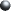Objective

This lab will demonstrate the transmission of a digital signal in the presence of noise. To accomplish this, a digital signal will be simulated, and a noise signal will be simulated. Then the two signal will be added and the signal will be decoded and bit-errors will be counted. The number of bit-errors will then be compared to an expected valued generated using "Q" functions. Various methods of digital modulation will be employed to show the advantages and disadvantages of various modulation techniques.Components Used

1) PC with Octave installed.Procedures

1) Simulate a plain digital signal in the presence of noise by using the command "digital(sd,M)" where sd is the standard deviation and M is the expansion factor.

2) Use 1 for sd and 4 for M and record the results.

3) Repeat step 2 using 8 and 16 for M.

4) Repeat steps 1 through 3 using sd = 2, 4.

5) Using the "ook(sd,M)" command, simulate an OOK modulated digital signal.

6) Simulate the signal using sd = 1, 2, 4 M=4.

7) Create a .m file that will simulate a signal using BPSK modulation.

8) Simulate a BPSK signal using M=8, sd=1, 2, 4.

9) Repeat steps 6 and 7 using QPSK modulation.Lab Data / Results

1) Table 1: Bit Error Rates:

Modulation Technique

M

RMS Noise Voltage

Expected BER

Observed BER

% Error

TTL

4

1v

.006

.008

33.3%

TTL

8

1v

.006

.008

33.3%

TTL

16

1v

.006

.008

33.3%

TTL

4

2v

.106

.100

5.66%

TTL

8

2v

.106

.111

4.72%

TTL

16

2v

.106

.087

17.9%

TTL

4

4v

.266

.278

4.51%

TTL

8

4v

.266

.263

1.13%

TTL

16

4v

.266

.253

4.89%

OOK

4

1v

.000

.000

0%

OOK

4

2v

.039

.052

33.3%

OOK

4

4v

.188

.163

13.3%

BPSK

4

1v

.000

.000

0%

BPSK

4

2v

.000

.000

0%

BPSK

4

4v

.039

.012

69.2%

QPSK

8

1v

.000

.000

0%

QPSK

8

2v

.000

.000

0%

QPSK

8

4v

.012

.017

41.7%

2) Diagram 1: Original TTL signal for M=4 sd = 1 digital transmission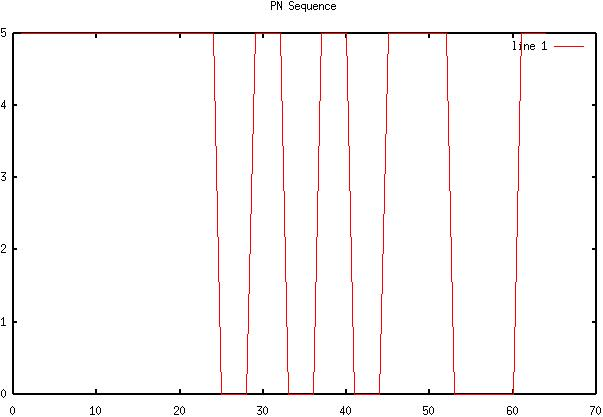3) Diagram 2: Digital transmission with noise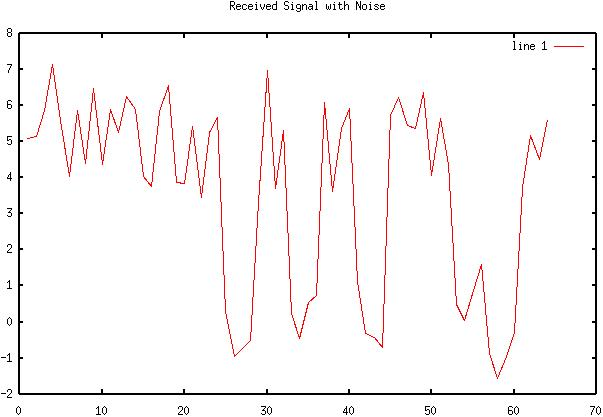4) Diagram 3: Recovered signal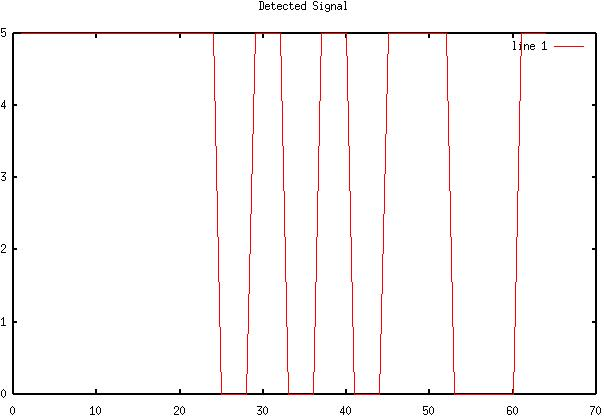5) Diagram 4: Original TTL signal for M=8 sd = 1 digital transmission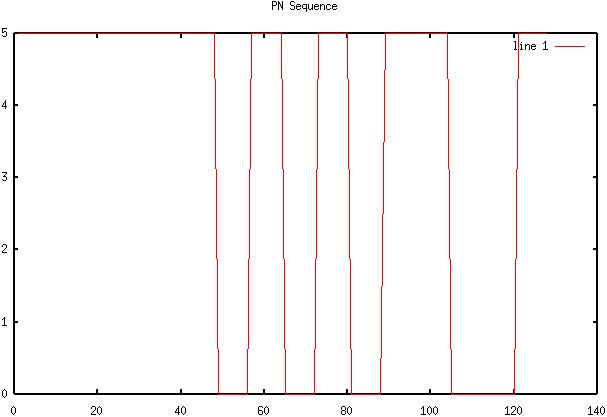6) Diagram 5: Digital transmission with noise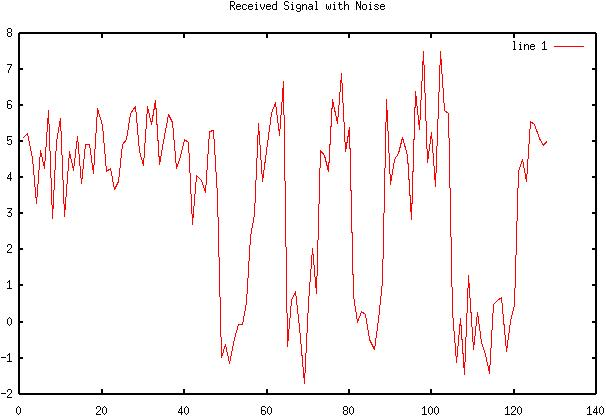7) Diagram 6: Recovered signal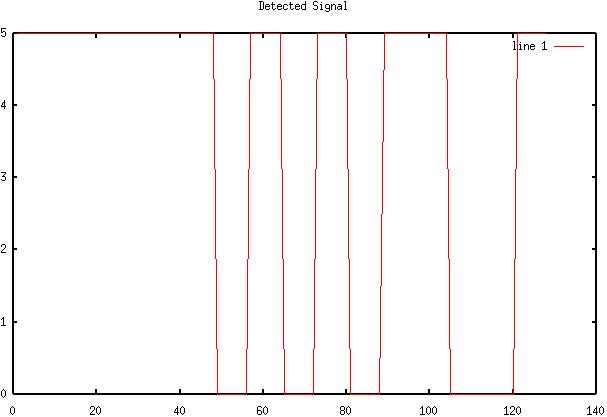8) Diagram 7: Original TTL signal for M=16 sd = 1 digital transmission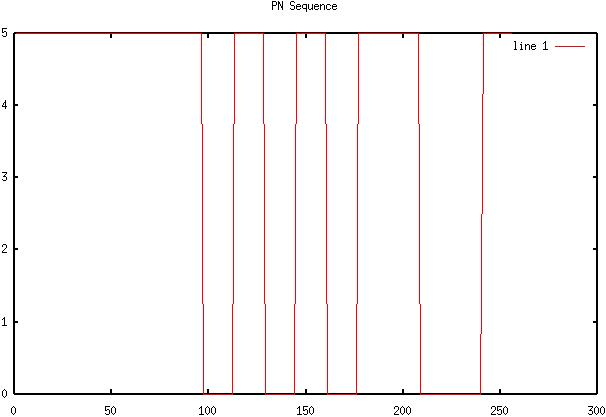9) Diagram 8: Digital transmission with noise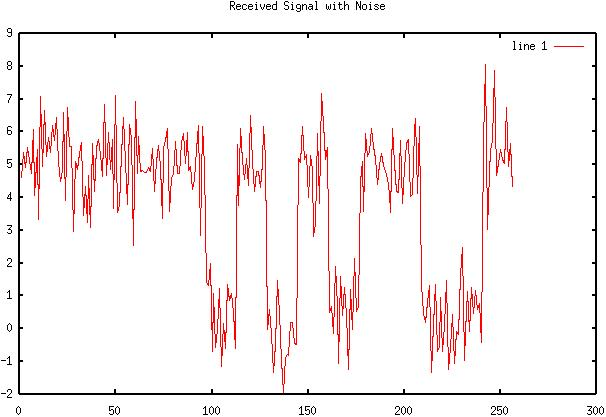10) Diagram 9: Recovered signal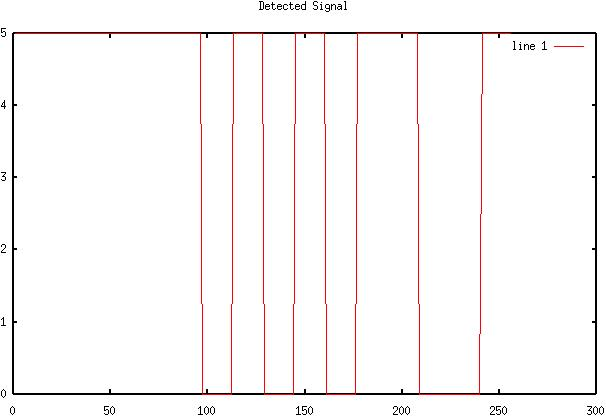11) Diagram 10: Original TTL signal for M=4 sd = 2 digital transmission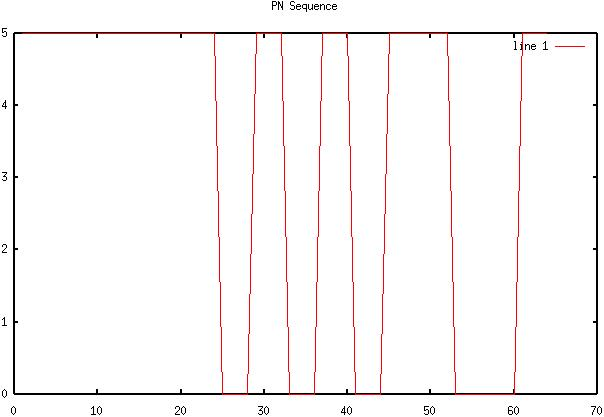12) Diagram 11: Digital transmission with noise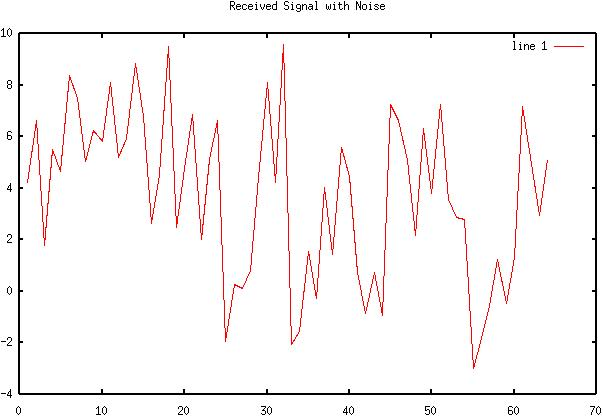13) Diagram 12: Recovered signal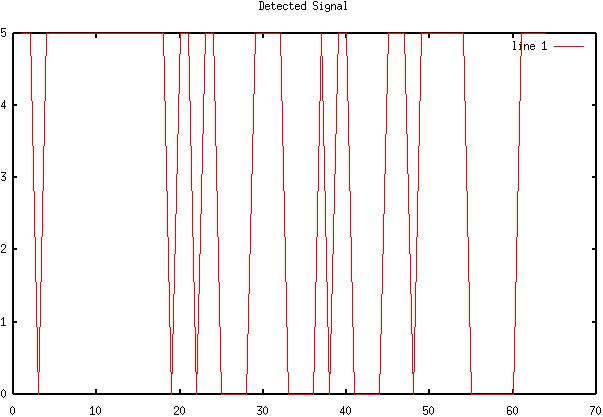14) Diagram 13: Original TTL signal for M=8 sd = 2 digital transmission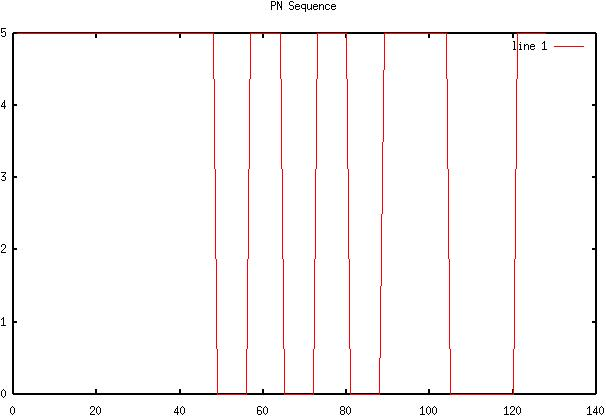15) Diagram 14: Digital transmission with noise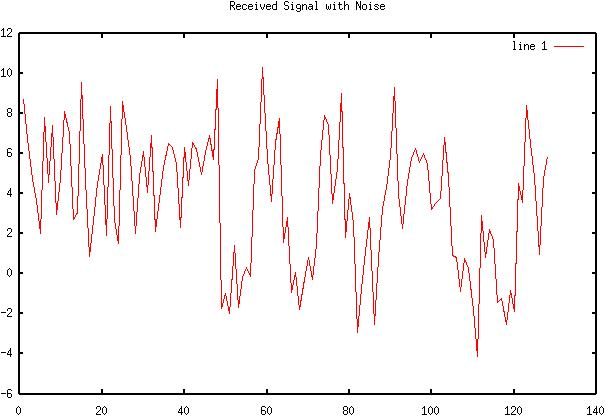16) Diagram 15: Recovered signal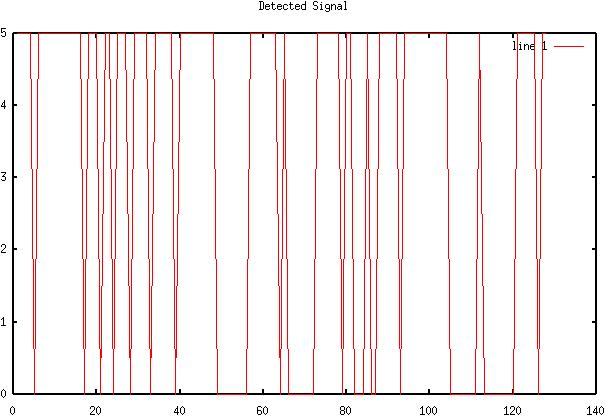17) Diagram 16: Original TTL signal for M=16 sd = 2 digital transmission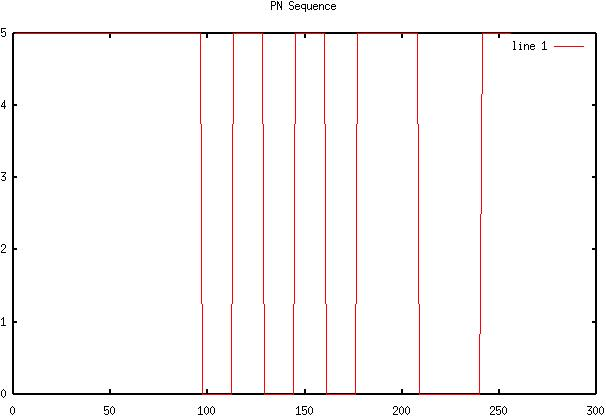18) Diagram 17: Digital transmission with noise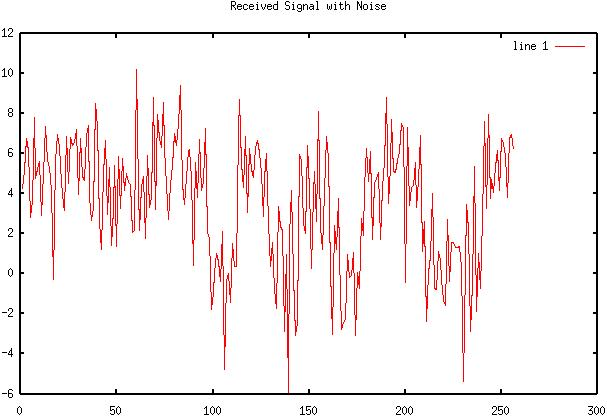19) Diagram 18: Recovered signal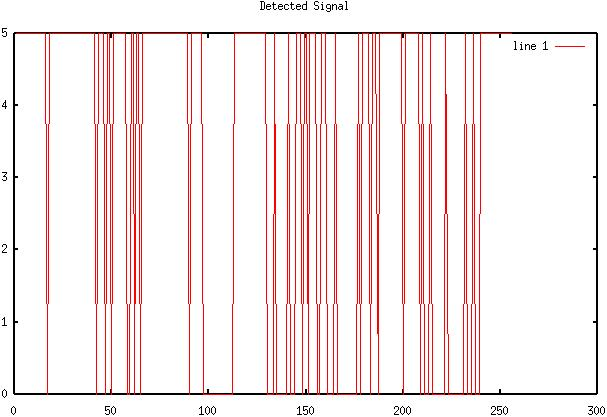20) Diagram 19: Original TTL signal for M=4 sd = 4 digital transmission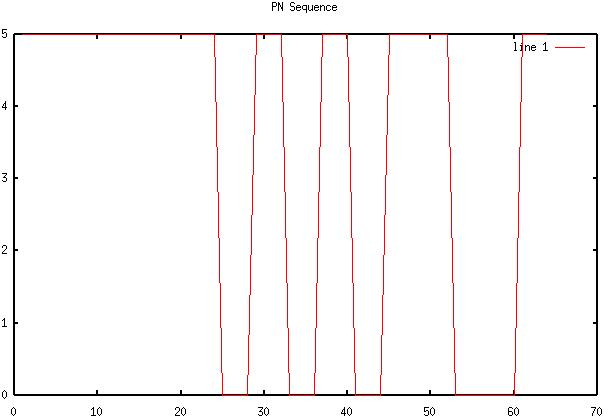21) Diagram 20: Digital transmission with noise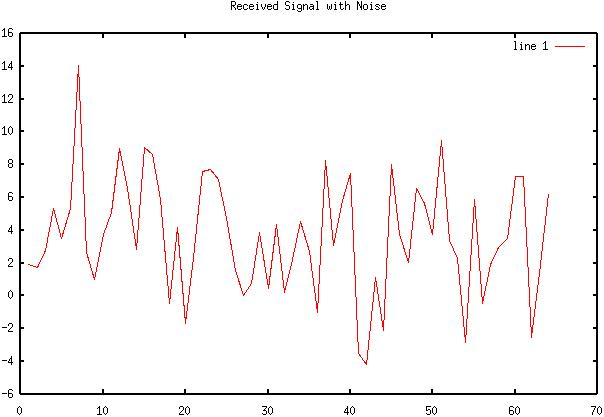22) Diagram 21: Recovered signal23) Diagram 22: Original TTL signal for M=8 sd = 4 digital transmission24) Diagram 23: Digital transmission with noise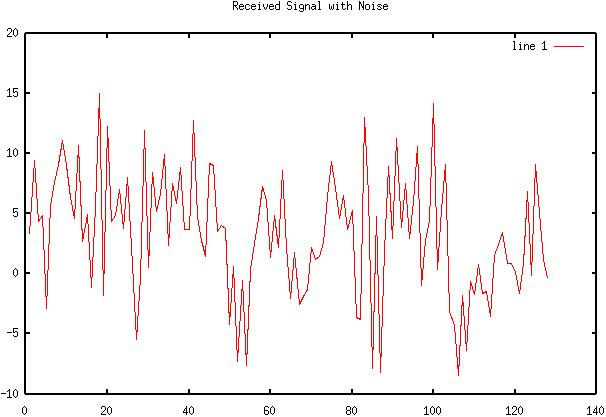25) Diagram 24: Recovered signal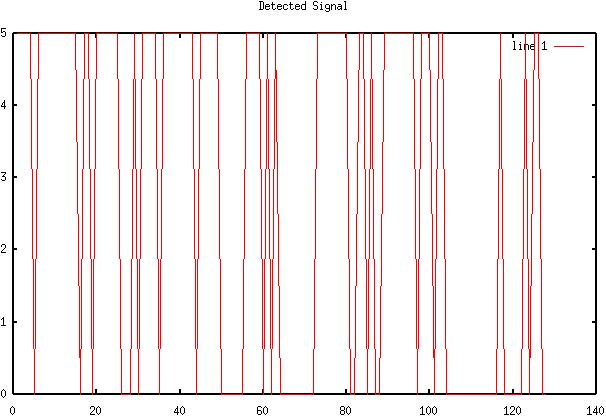26) Diagram 25: Original TTL signal for M=16 sd = 4 digital transmission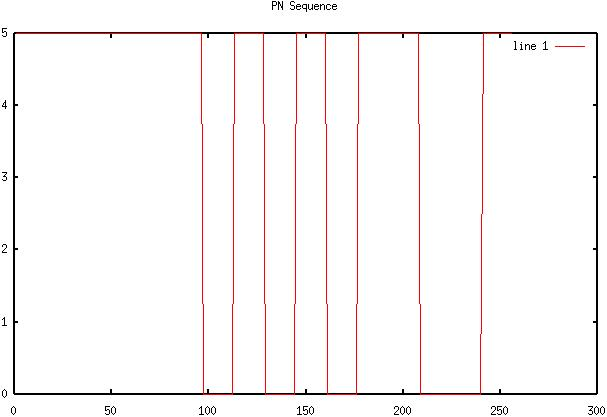27) Diagram 26: Digital transmission with noise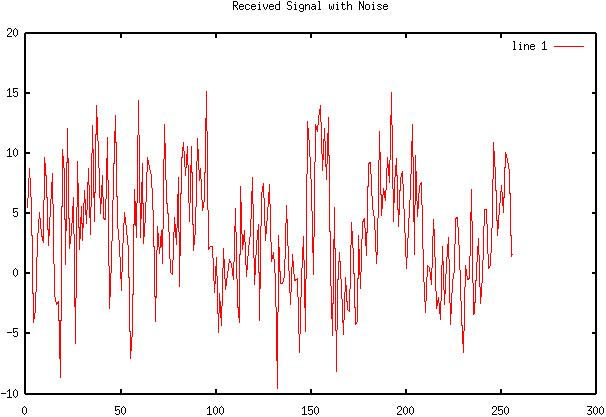28) Diagram 27: Recovered signal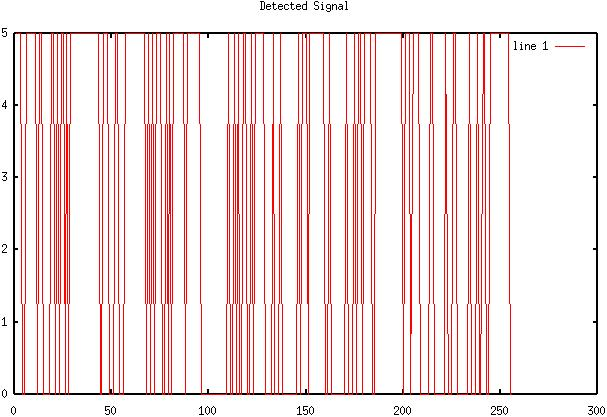29) Diagram 28: Original TTL signal for M=4 sd = 1 OOK transmission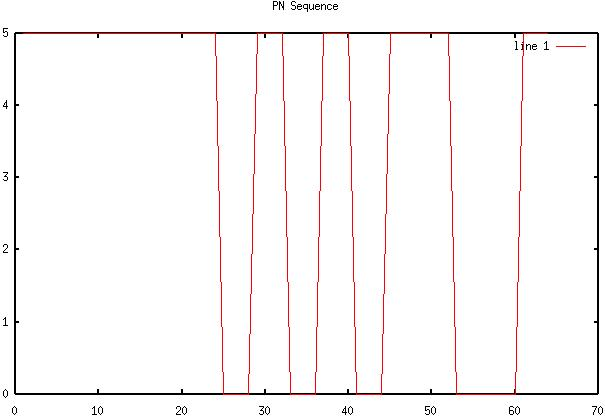30) Diagram 29: OOK Signal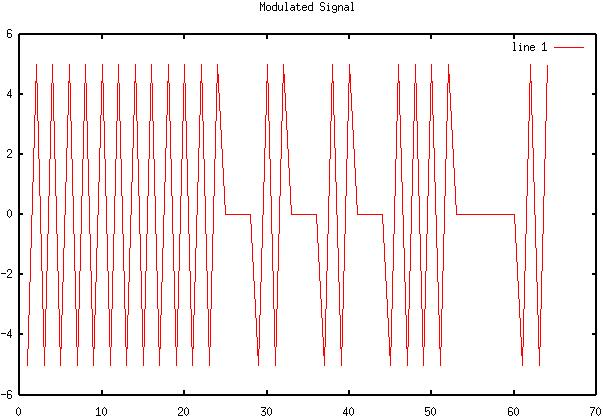31) Diagram 30: OOK Signal and noise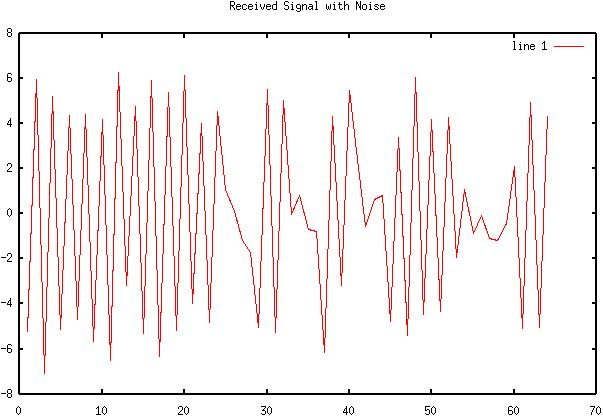32) Diagram 31: OOK Signal after mixing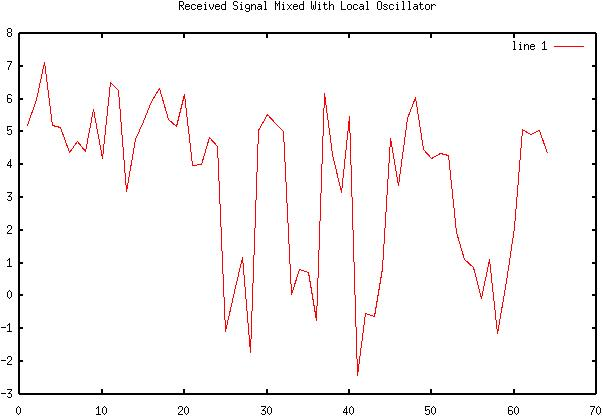33) Diagram 32: Integrated and dumped signal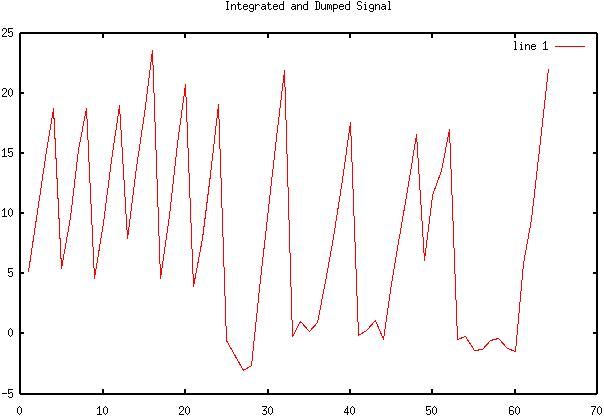34) Diagram 33: Original TTL signal for M=4 sd = 2 OOK transmission35) Diagram 34: OOK Signal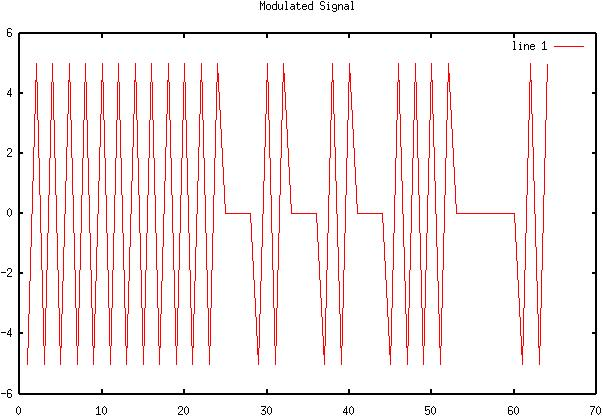36) Diagram 35: OOK Signal and noise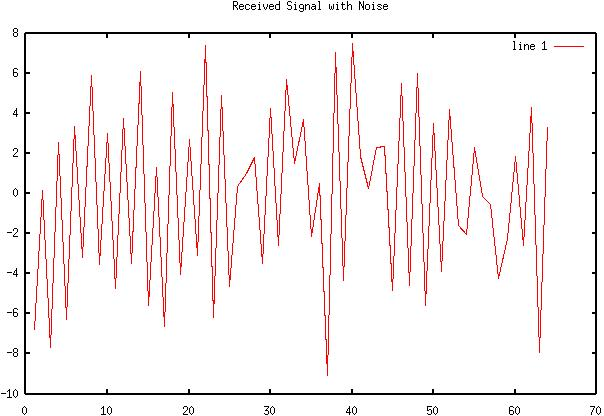37) Diagram 36: OOK Signal after mixing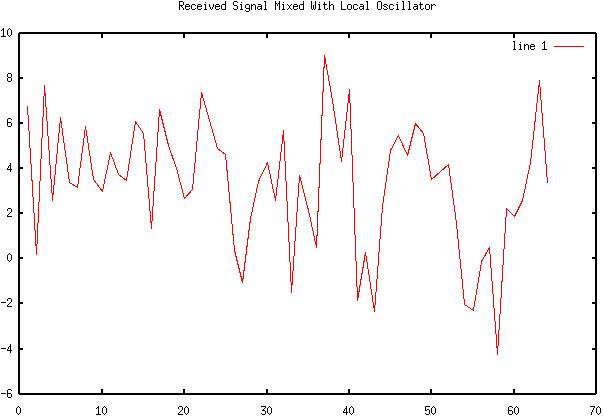38) Diagram 37: Integrated and dumped signal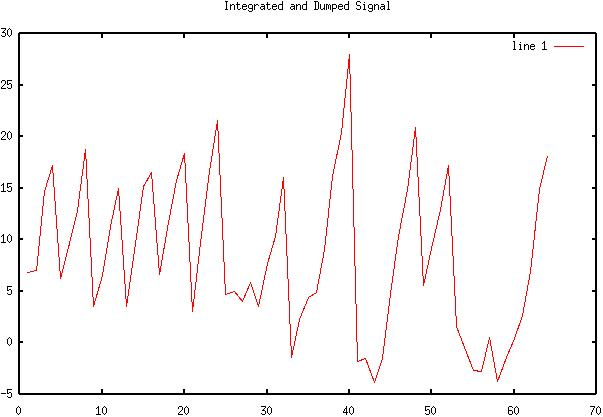39) Diagram 38: Original TTL signal for M=4 sd = 4 OOK transmission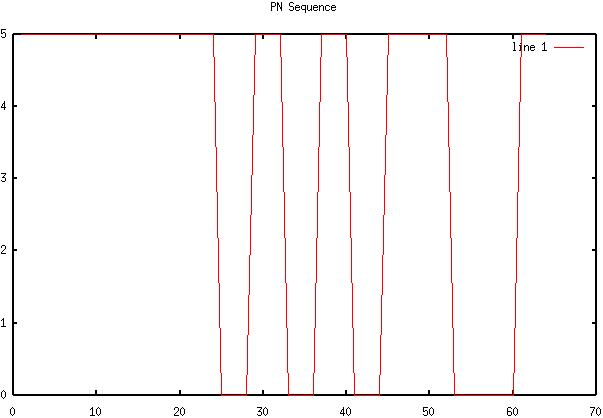40) Diagram 39: OOK Signal41) Diagram 40: OOK Signal and noise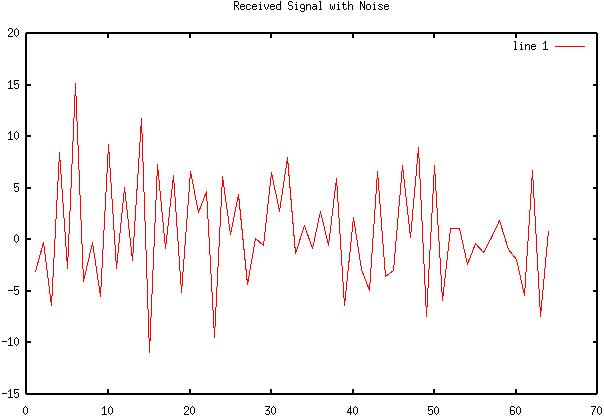42) Diagram 41: OOK Signal after mixing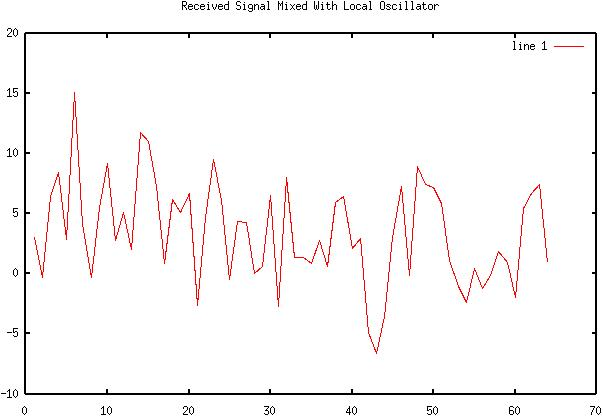43) Diagram 42: Integrated and dumped signal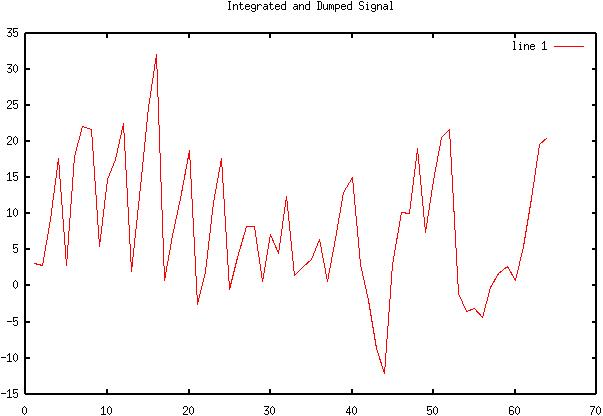44) Diagram 43: Original TTL signal for M=4 sd = 1 BPSK transmission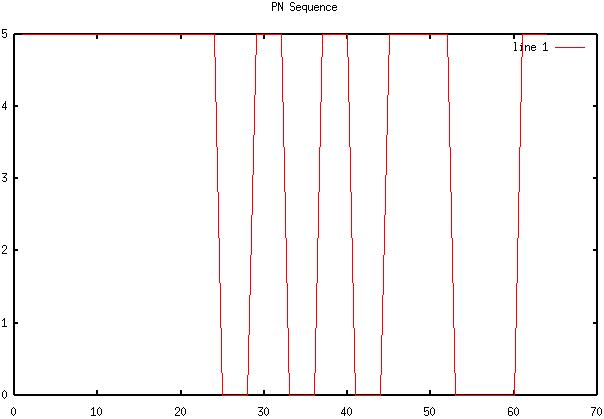45) Diagram 44: BPSK Signal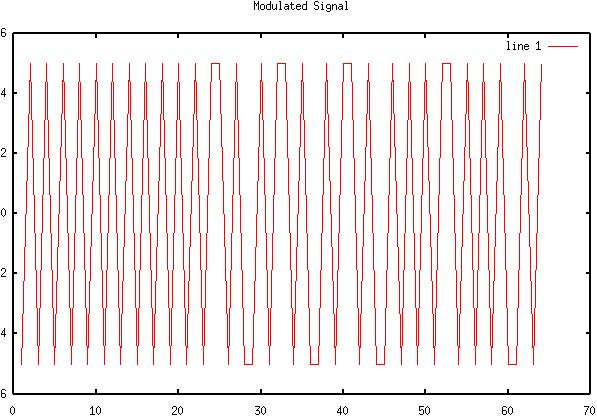46) Diagram 45: BPSK Signal and noise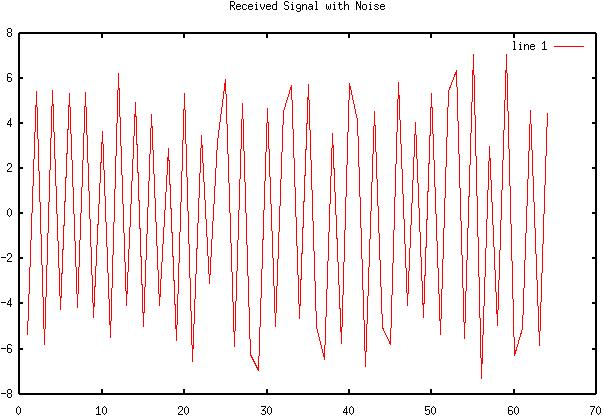47) Diagram 46: BPSK Signal after mixing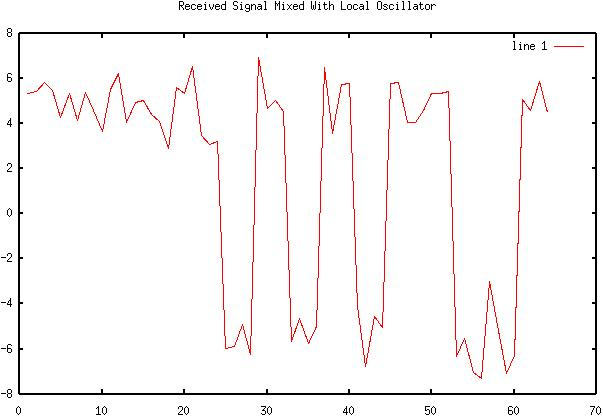48) Diagram 47: Integrated and dumped signal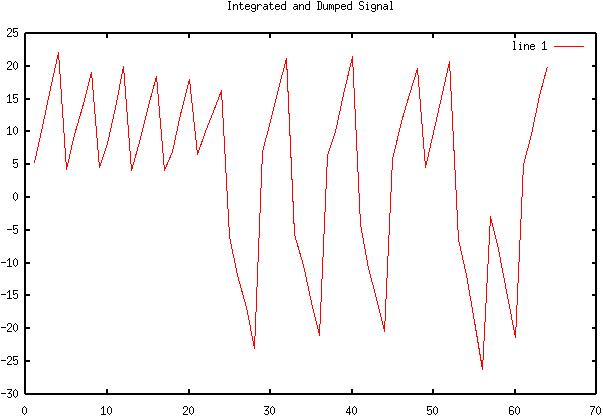49) Diagram 48: Final received signal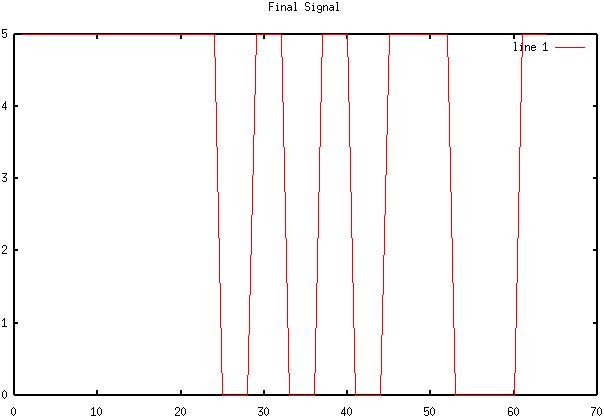50) Diagram 49: Original TTL signal for M=4 sd = 2 BPSK transmission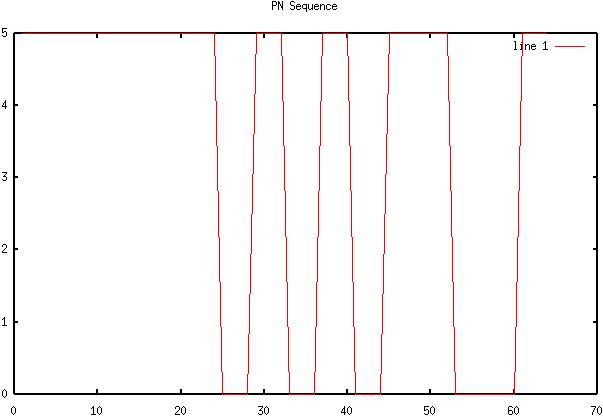51) Diagram 50: BPSK Signal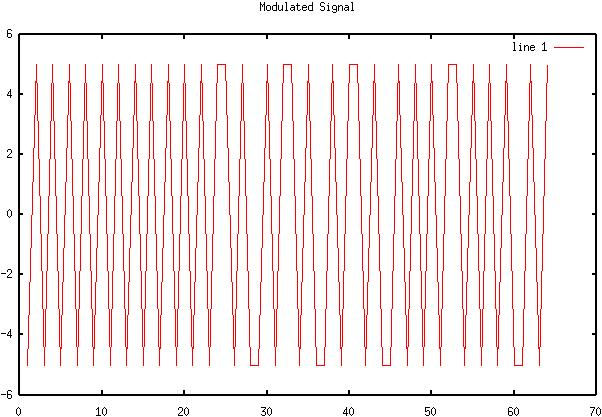52) Diagram 51: BPSK Signal and noise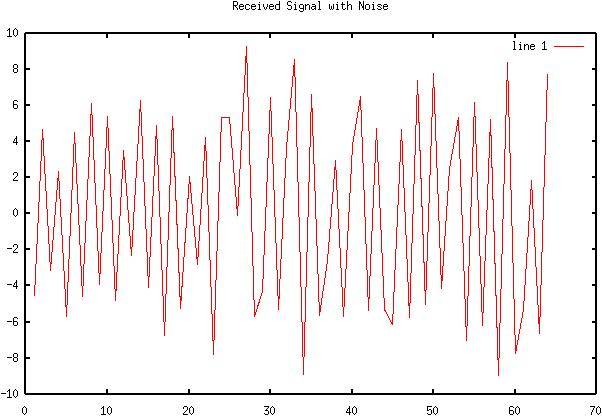53) Diagram 52: BPSK Signal after mixing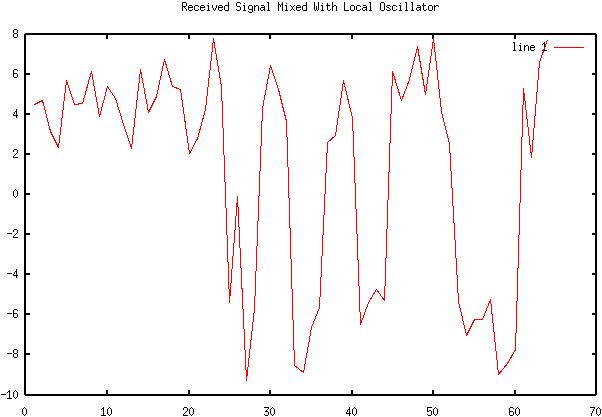54) Diagram 53: Integrated and dumped signal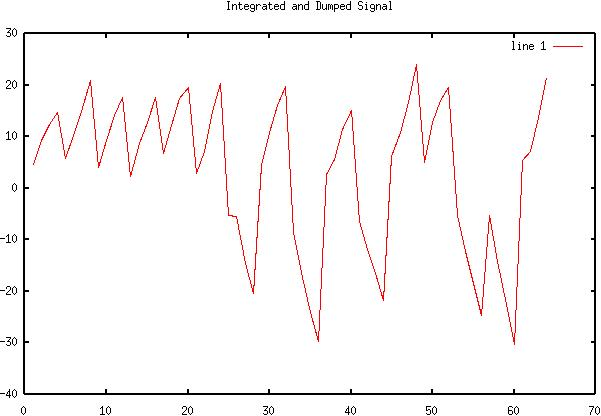55) Diagram 54: Final received signal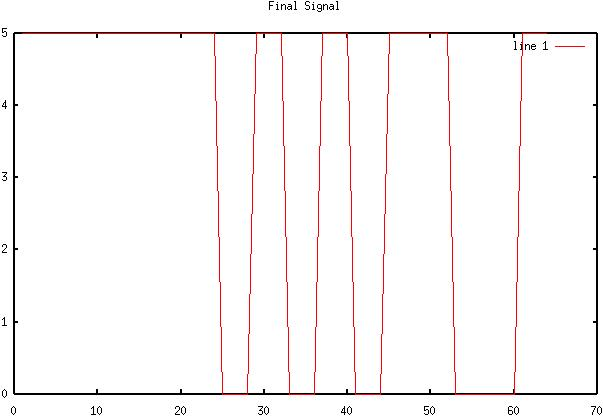56) Diagram 55: Original TTL signal for M=4 sd = 4 BPSK transmission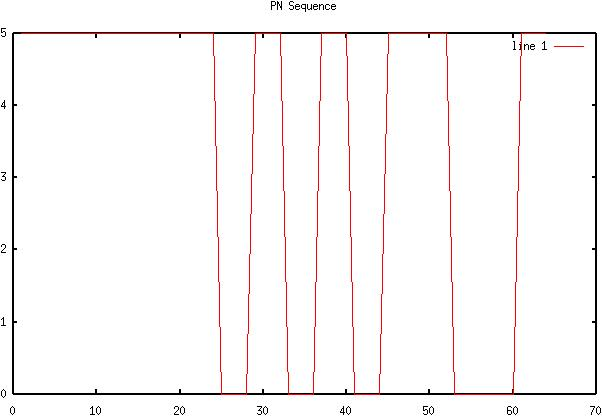57) Diagram 56: BPSK Signal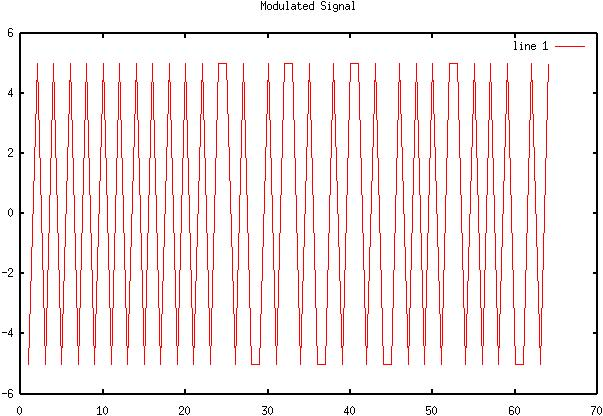58) Diagram 57: BPSK Signal and noise59) Diagram 58: BPSK Signal after mixing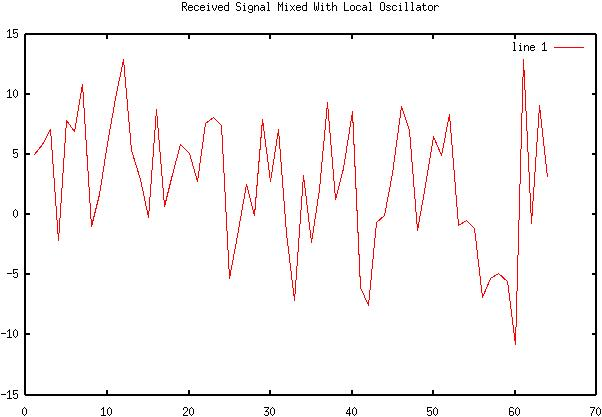60) Diagram 59: Integrated and dumped signal61) Diagram 60: Final received signal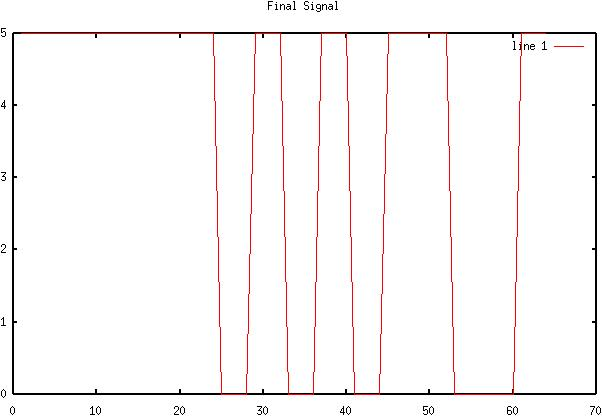62) Diagram 61: Original TTL signal for M=8 sd = 1 QPSK transmission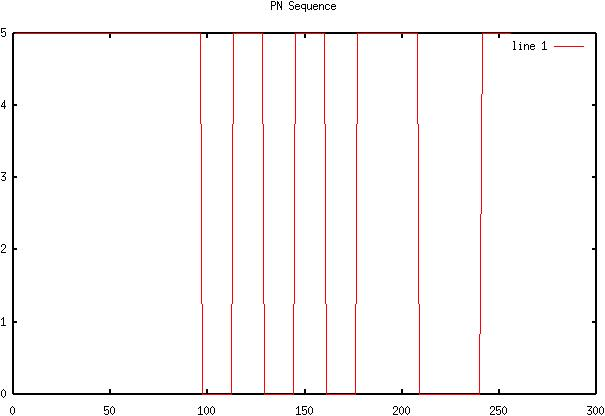63) Diagram 62: QPSK Signal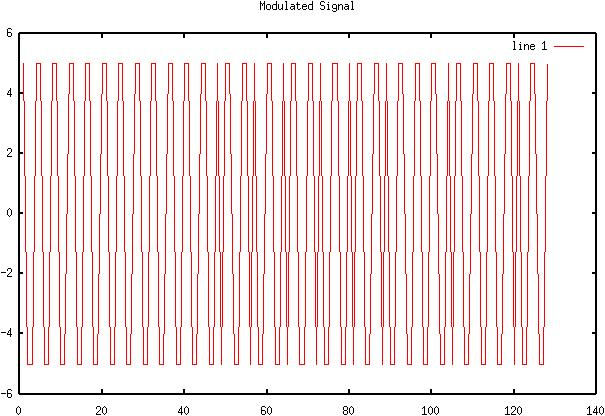64) Diagram 63: QPSK Signal and noise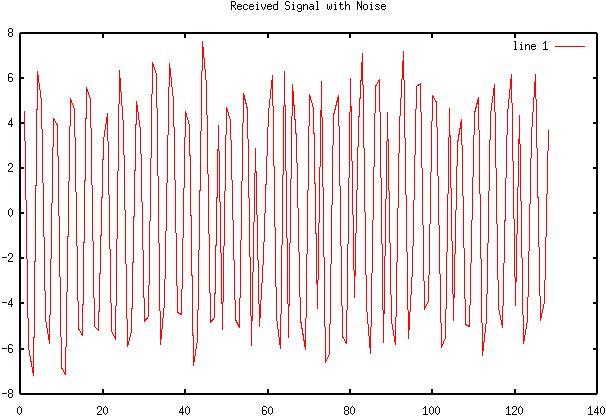65) Diagram 64: QPSK Signal after mixing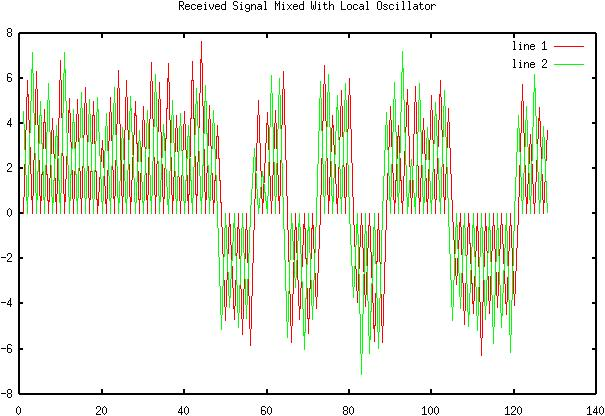66) Diagram 65: Integrated and dumped signal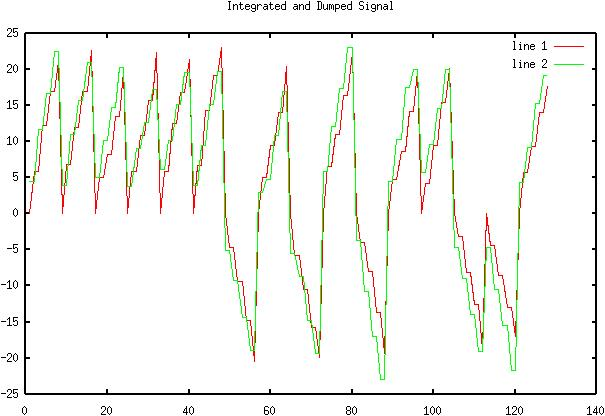67) Diagram 66: Final received signal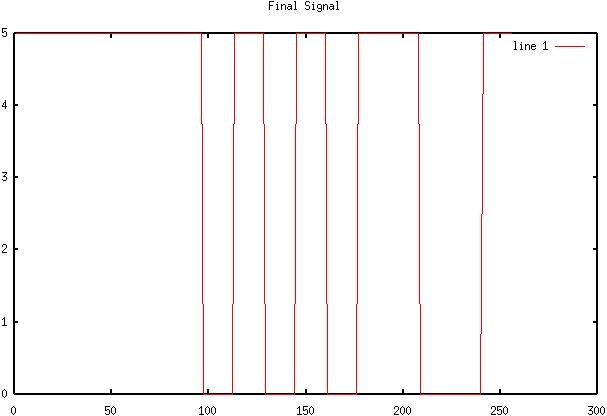68) Diagram 67: Original TTL signal for M=8 sd = 2 QPSK transmission69) Diagram 68: QPSK Signal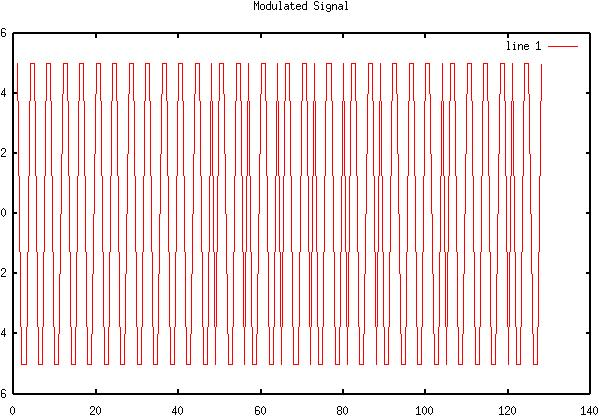70) Diagram 69: QPSK Signal and noise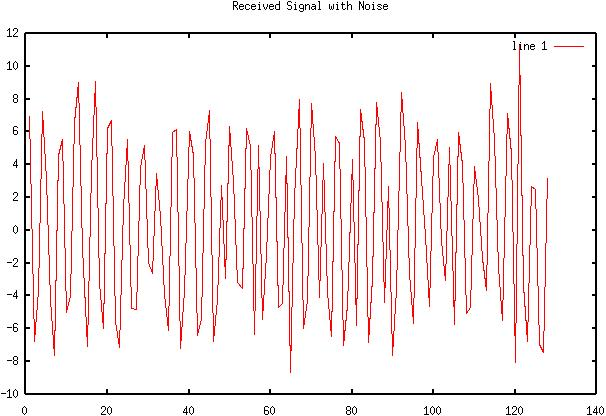71) Diagram 70: QPSK Signal after mixing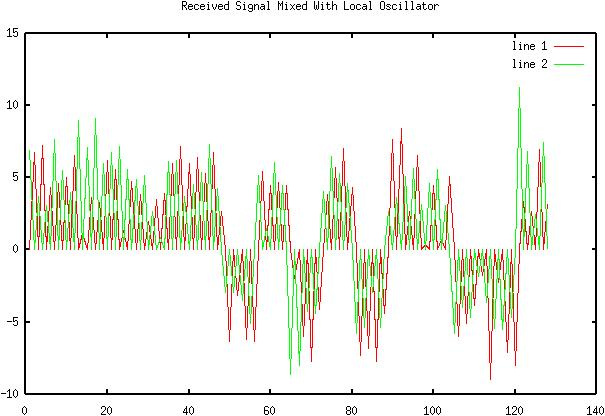72) Diagram 71: Integrated and dumped signal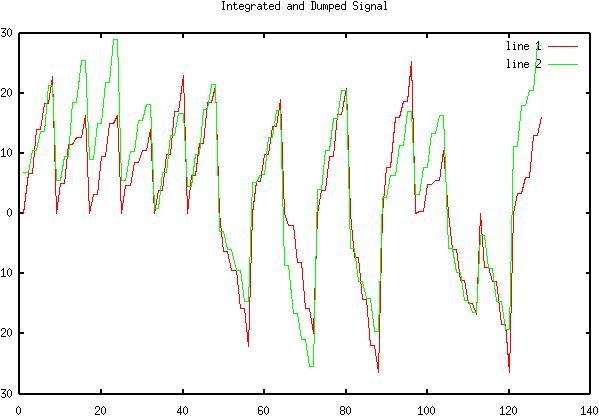73) Diagram 72: Final received signal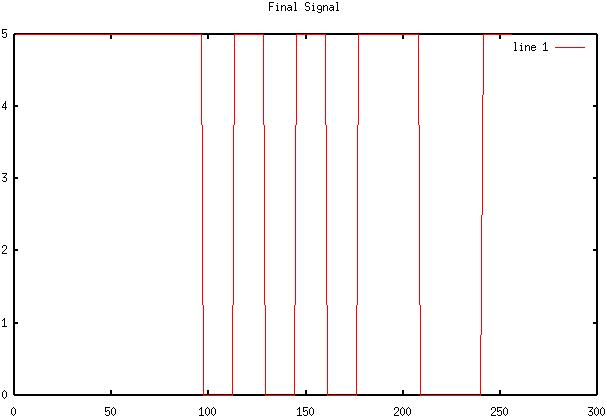74) Diagram 73: Original TTL signal for M=8 sd = 4 QPSK transmission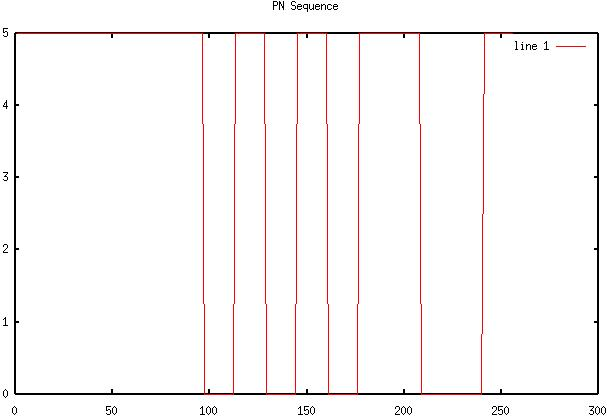75) Diagram 74: QPSK Signal76) Diagram 75: QPSK Signal and noise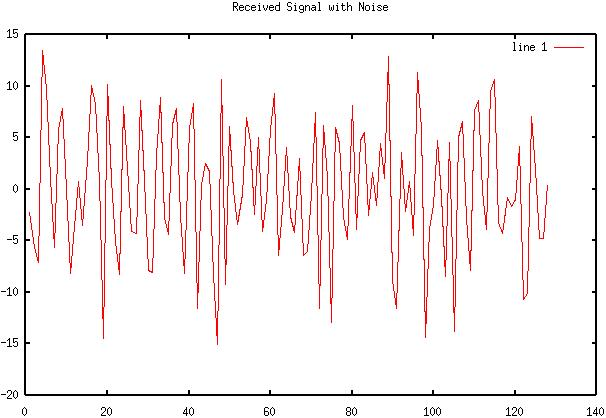77) Diagram 76: QPSK Signal after mixing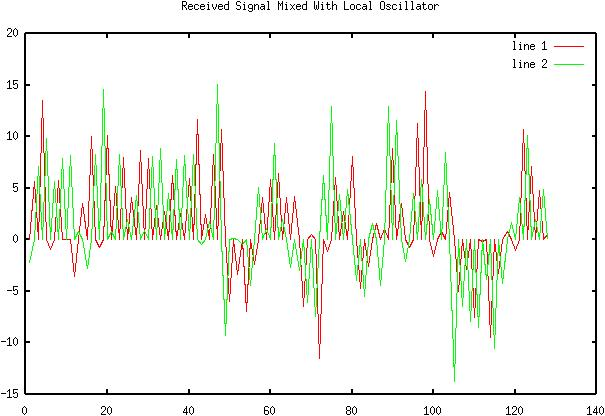78) Diagram 77: Integrated and dumped signal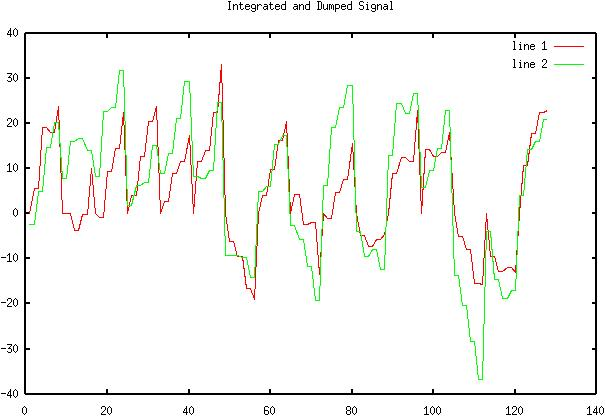79) Diagram 78: Final received signal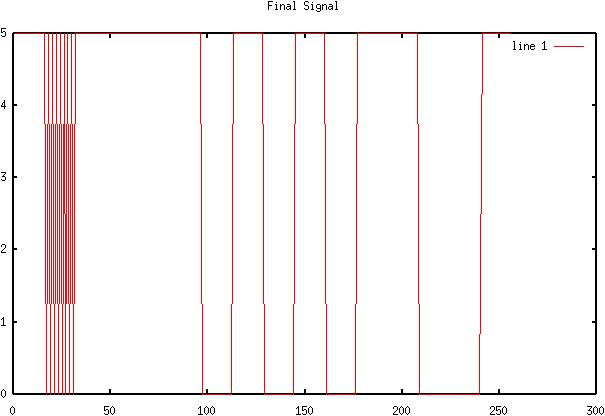Conclusions

This lab has demonstrated how various forms of digital signaling are affected by noise in the system. The results are fairly accurate, even though some of the percent errors are high. For QPSK modulation it was decided that the scaling factor (M value) should be set to 8. For some reason, when sampling a sine wave, as opposed to a cosine wave, the signal was indistinguishable. As a result, half of the bits were dropped before demodulation. To make sure the probability of a bit error was correct, several other tests were made using "Tesla coil" noise levels (sd > 1000). The result was usually .5 or something close to that value, which is expected.Attachments

1. Original lab handout

2. Original lab data

3. Calculations

4. Octave log

5. BPSK .m file

6. QPSK .m file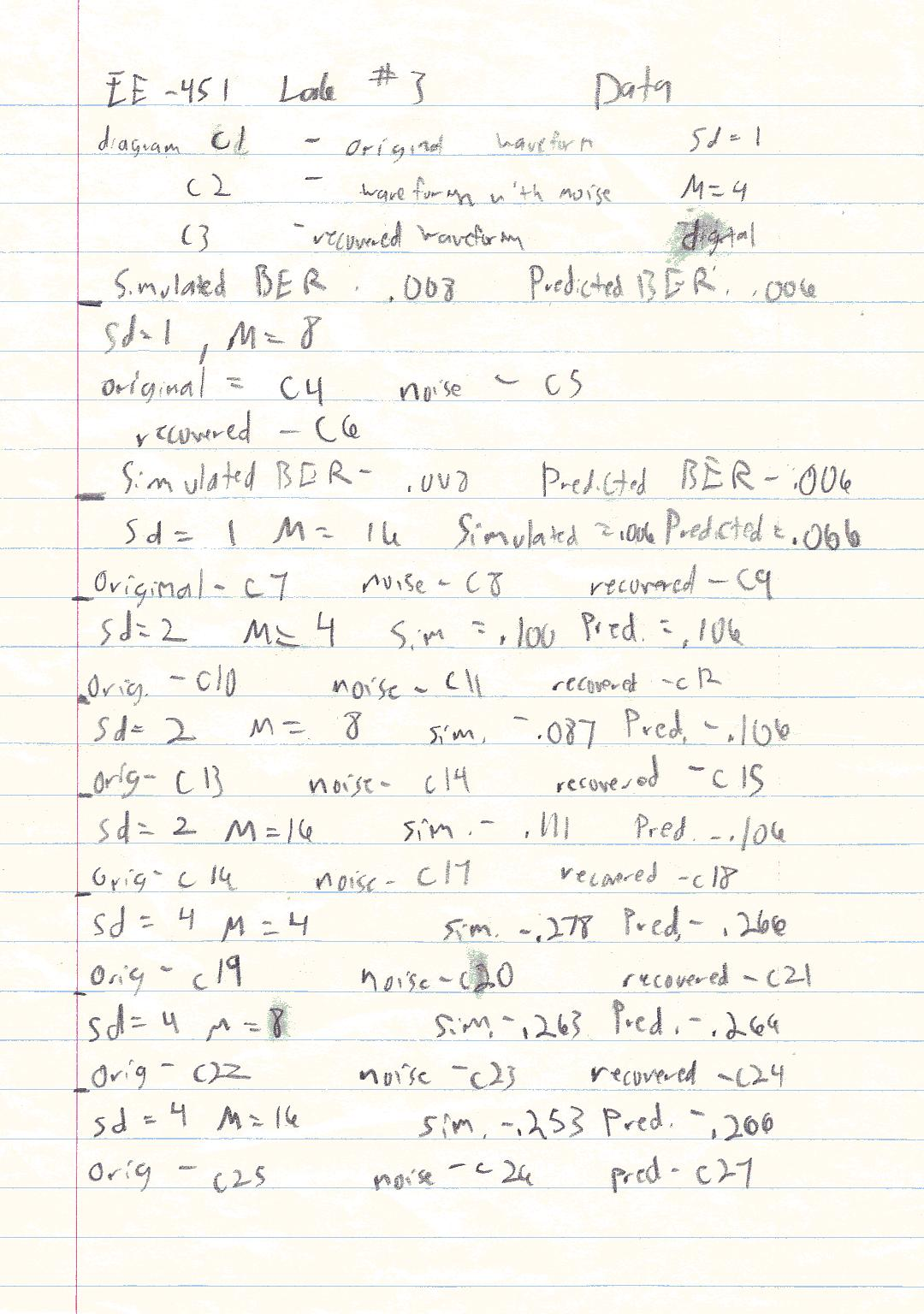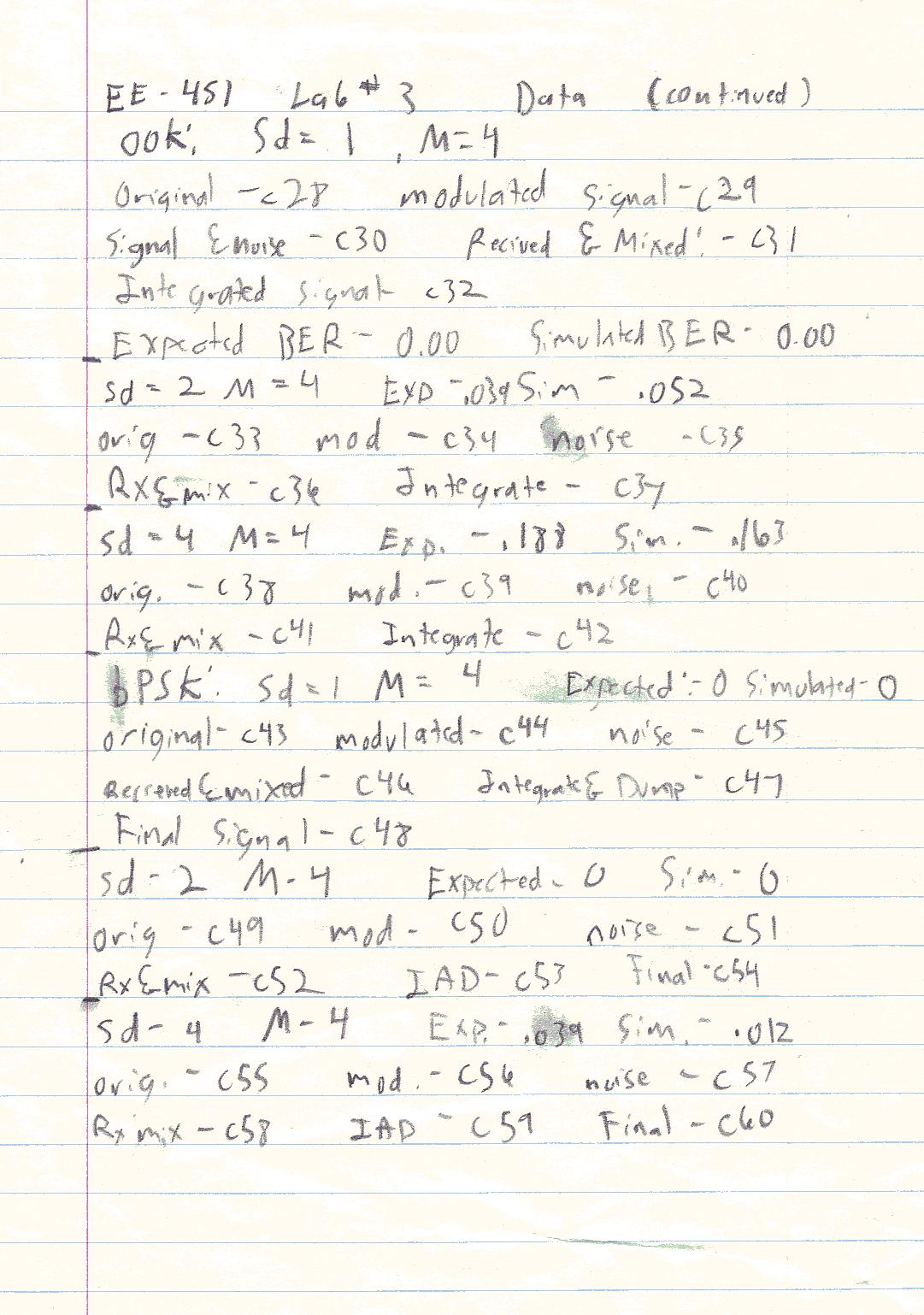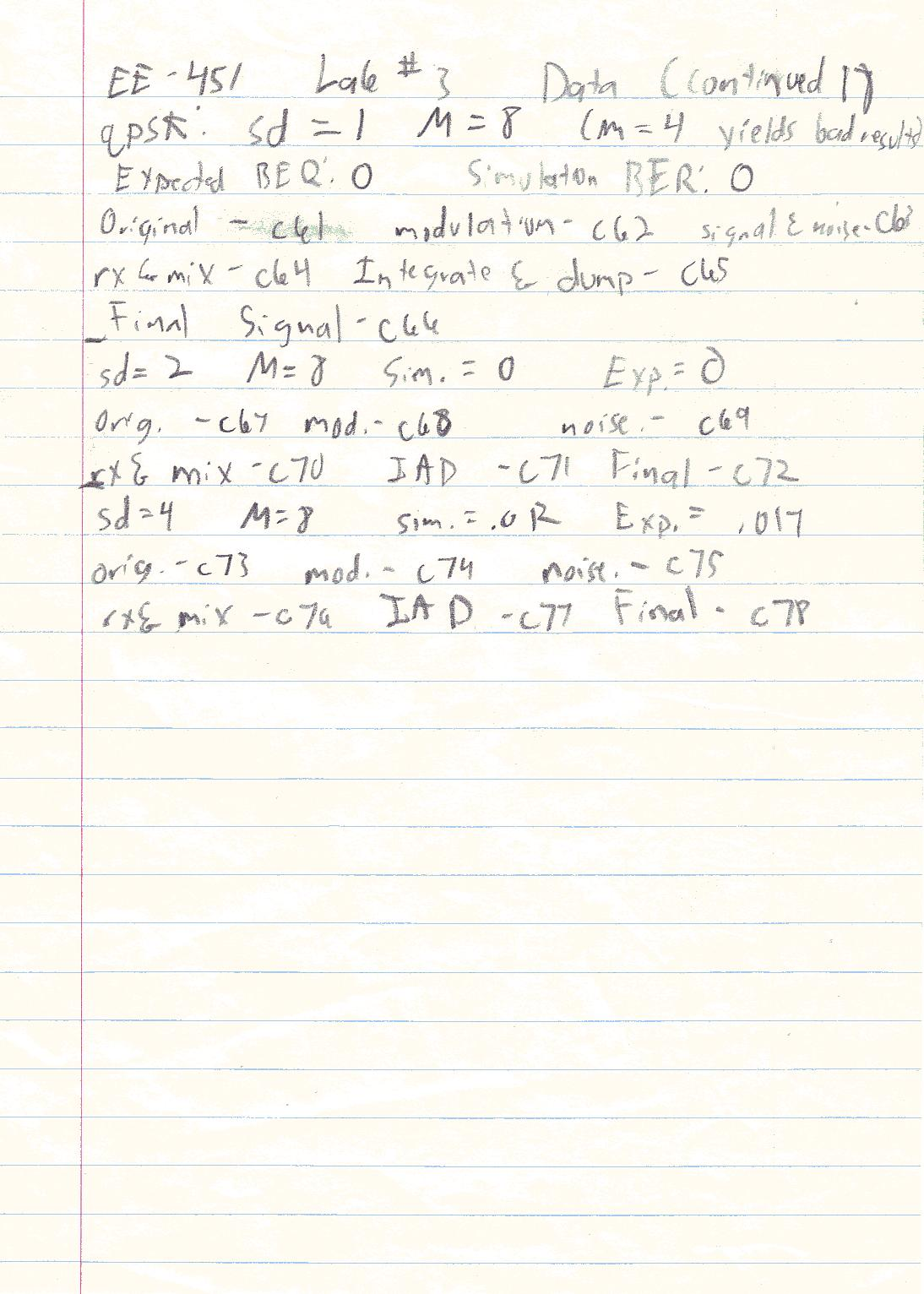GNU Octave, version 2.0.17 (i686-pc-linux-gnu).

Copyright (C) 1996, 1997, 1998, 1999, 2000, 2001, 2002 John W. Eaton.

This is free software with ABSOLUTELY NO WARRANTY.

For details, type `warranty'.

octave:1> digital(2.5,4)

warning: function `digital' defined within script file `/home/Leeloo/lab3/digital.m'

octave:2> digital(2.5,4)

warning: function `prs63' defined within script file `/home/Leeloo/lab3/prs63.m'error: value on right hand side of assignment is undefined

error: evaluating assignment expression near line 27, column 3

error: called from `digital'

octave:2> digital(2.5,4)

warning: function `prs' defined within script file `/home/Leeloo/lab3/prs.m'

error: value on right hand side of assignment is undefined

error: evaluating assignment expression near line 12, column 4

error: called from `prs63'

error: called from `digital'

octave:2> digital(2.5,4)

warning: ignoring trailing garbage after end of function

near line 5 of file `mod.m'

warning: function `noise' defined within script file `/home/Leeloo/lab3/noise.m'error: value on right hand side of assignment is undefined

error: evaluating assignment expression near line 32, column 3

error: called from `digital'

octave:2> digital(1,4)

warning: function `detect' defined within script file `/home/Leeloo/lab3/detect.m'

error: value on right hand side of assignment is undefined

error: evaluating assignment expression near line 38, column 4

error: called from `digital'

octave:2>

Display all 858 possibilities? (y or n)

octave:2> digital(1,4)

octave:2> digital(1,4)

warning: function `ber' defined within script file `/home/Leeloo/lab3/ber.m'

error: value on right hand side of assignment is undefined

error: evaluating assignment expression near line 43, column 4

error: called from `digital'

octave:2> digital(1,4)

Bit error rate from simulation = 0.013

warning: function `q' defined within script file `/home/Leeloo/lab3/q.m'

error: value on right hand side of assignment is undefined

error: evaluating assignment expression near line 47, column 7

error: evaluating if command near line 46, column 1

error: called from `digital'

octave:2> digital(1,4)

Bit error rate from simulation = 0.008

Predicted bit error rate = 0.006

octave:3> digital(1,8)

Bit error rate from simulation = 0.008

Predicted bit error rate = 0.006

octave:4> digital(1,16)

Bit error rate from simulation = 0.006

Predicted bit error rate = 0.006

octave:5> digital(2,4)

Bit error rate from simulation = 0.100

Predicted bit error rate = 0.106

octave:6> digital(2,8)

Bit error rate from simulation = 0.087

Predicted bit error rate = 0.106

octave:7> digital(2,16)

Bit error rate from simulation = 0.111

Predicted bit error rate = 0.106

octave:8> digital(4,4)

Bit error rate from simulation = 0.278

Predicted bit error rate = 0.266

octave:9> digital(4,8)

Bit error rate from simulation = 0.263

Predicted bit error rate = 0.266

octave:10> digital(4,16)

Bit error rate from simulation = 0.253

Predicted bit error rate = 0.266

octave:11> ook(1,4)

warning: function `ook' defined within script file `/home/Leeloo/lab3/ook.m'

octave:12> ook(1,4)

error: value on right hand side of assignment is undefined

error: evaluating assignment expression near line 51, column 4

error: called from `ook'

octave:12> ook(1,4)

warning: function `sam' defined within script file `/home/Leeloo/lab3/sam.m'

error: value on right hand side of assignment is undefined

error: evaluating assignment expression near line 56, column 4

error: called from `ook'

octave:12> ook(1,4)

warning: function `expand' defined within script file `/home/Leeloo/lab3/expand.m'

error: value on right hand side of assignment is undefined

error: evaluating assignment expression near line 58, column 4

error: called from `ook'

octave:12> ook(1,4)

Bit error rate from simulation = 0.000

Predicted bit error rate = 0.000

octave:13> ook(2,4)

Bit error rate from simulation = 0.052

Predicted bit error rate = 0.188

octave:15> bpsk(1,4)

warning: function `bpsk' defined within script file `/home/Leeloo/lab3/bpsk.m'

octave:16> bpsk(1,4)

Bit error rate from simulation = 0.000

Predicted bit error rate = 0.000

octave:17> bpsk(2,4)

Bit error rate from simulation = 0.000

Predicted bit error rate = 0.000

octave:18> bpsk(4,4)

Bit error rate from simulation = 0.012

Predicted bit error rate = 0.039

octave:19> qpsk(1,8)

warning: function `qpsk' defined within script file `/home/Leeloo/lab3/qpsk.m'

octave:20> qpsk(1,8)

warning: ignoring trailing garbage after end of function

near line 5 of file `mod.m'

Bit error rate from simulation = 0.000

Predicted bit error rate = 0.000

octave:21> qpsk(2,8)

Bit error rate from simulation = 0.000

Predicted bit error rate = 0.000

octave:22> qpsk(4,8)

Bit error rate from simulation = 0.012

Predicted bit error rate = 0.017

octave:23>